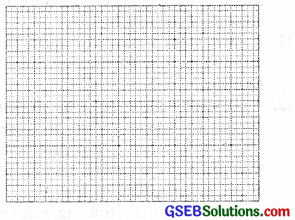# GSEB Class 6 Maths Notes Chapter 10 Mensuration

This GSEB Class 6 Maths Notes Chapter 10 Mensuration covers all the important topics and concepts as mentioned in the chapter.

## Mensuration Class 6 GSEB Notes

Introduction:
We have learn about a point, a line and line segment. The line segment whether plane or curved form plane figures. When we talk about some plane figures, we think of their regions and their boundaries. So we need some measure to compare them. In this chapter, we shall learn about the concept of perimeter and area of plane figures.

Perimeter:
The length of the boundary is known as the perimeter of the figure.
Perimeter of a figure is the total length of its boundary.
Perimeter of a figure = Some of length of its all sides.

1. Perimeter of a Triangle.
Perimeter of a triangle = Sum of the lengths of its three sides. Perimeter of an equilateral triangle = 3 × (length of its side)

2. Perimeter of a Rectangle.
Perimeter of a rectangle. = length + breadth + length + breadth = 2 × (length) + 2 × (breadth)
= 2 × (length + breadth)

Also remember these :
Length of rectangle = $$\frac{1}{2}$$ perimeter – breadth.
Breadth of rectangle 1 = $$\frac{1}{2}$$ perimeter – length.

3. Perimeter of a Square.
Perimeter of square = 4 × side
Perimeter of square Side of square = $$\frac{\text { Perimeter of square }}{4}$$

4. Perimeter of a Regular Pentagon.
A regular pentagon is a polygon with 5 equal sides.
Perimeter of a Pentagon = 5 × Length of a side of regular pentagon
⇒ Length of a side of a regular pentagon = $$\frac{\text { Perimeter of a regular pentagon }}{5}$$

5. Perimeter of a Regular Hexagon.
A regular hexagon is a polygon with 6 equal sides.
∴ Perimeter of a hexagon = 6 × Length of a side of regular hexagon
⇒ Length of a side of a regular Hexagon = $$\frac{\text { Perimeter of a regular hexagon }}{6}$$Area:
The magnitude of the portion of the plane enclosed or covered in a simple closed region/figure is known as the area of this figure.
The measurement of the region enclosed by a close plane figure is called its area.

Unit of Area:
Let a square of side 1 unit. It covers the region 1 square units. So we always denote the area in square units.

Finding Area by the use of squared paper (Graph paper) :
The squared paper or a graph paper is a convenient method for finding the approximate area of a region enclosed by any simple closed curve.

Finding Area by Counting Squares on the Squared Paper
The squared paper or a graph paper is a convenient method for finding the approximate area of a region enclosed by any simple closed curve.The following rules are used to find area by counting Squares:

• Rule 1: Count the number of complete squares enclosed by the figure. Let the number of these complete squares be m.
• Rule 2: Count the number of exact half squares (length-wise, breadth¬wise, or diagonally.) Let the number of these exactly half squares be p.
• Rule 3: Count the number of squares more than half of whose portion is enclosed by the figure. Let the number of such squares be n.
• Rule 4: Ignore the squares that have less than half of their portion enclosed by the figure.
• Rule 5: Compute the number
A = (m + n + $$\frac{1}{2}$$p)

Measurement of Area of Using Formula
Area of rectangle = Length × Breadth
Length = $$\frac{\text { Area }}{\text { Breadth }}$$ or Breadth = $$\frac{\text { Area }}{\text { Length }}$$
Area of Square = side × side.